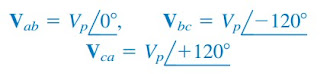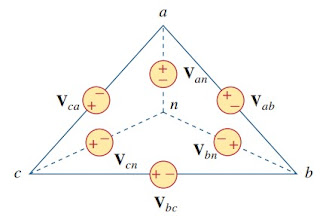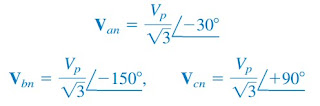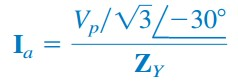omG8HI9LSKFW6lhCj8prTs0Z6lUhdOT9Jhi1Sf4m

# Three-Phase Electric Circuits: Balanced Delta-Wye Connection

A delta-wye system is a system with delta-connected sources with wye-connected loads.
A balanced delta-wye system consists of a balanced ∆-connected source feeding a balanced Y-connected load.
Before you start, it wise for you to read what is three-phase circuits first.

## Balanced Delta-Wye Connection

Consider the ∆-Y circuit in Figure.(1).Figure 1. A balanced ∆-Y connection
Again, assuming the abc sequence, the phase voltages of a delta-connected source are(1)
These are also the line voltages as well as the phase voltages.

We can obtain the line currents in many ways. One way is to apply KVL to loop a AN B b a in Figure.(1), writing(2)
But Ib lags Ia by 120◦since we assumed the abc sequence; that is, Ib = Ia ∠− 120◦. Hence,(3)
Substituting Equation.(3) into (2) gives(4)
From this, we obtain the other line currents Ib and Ic using the positive phase sequence, i.e., Ib = Ia ∠− 120◦, Ic = Ia ∠+ 120◦. The phase currents are equal to the line currents.Figure 2. Transforming a ∆-connected source to an equivalent Y-connected source.
Another way to obtain the line currents is to replace the delta connected source with its equivalent wye-connected source, as shown in Figure.(2). In balanced wye-wye connection, we found that the line-to-line voltages of a wye-connected source lead their corresponding phase voltages by 30◦.

Therefore, we obtain each phase voltage of the equivalent wye-connected source by dividing the corresponding line voltage of the delta-connected source by √3 and shifting its phase by −30◦. Thus, the equivalent wye connected source has the phase voltages(5)
If the delta-connected source has source impedance ZS per phase, the equivalent wye-connected source will have a source impedance of ZS/3 per phase.Figure 3. The single-phase equivalent circuit
Once the source is transformed into the wye, the circuit becomes a wye-wye system. Therefore, we can use the equivalent single-phase circuit is shown in Figure.(3), from which the line current for phase a is(6)
which is the same as Equation.(4)

Alternatively, we may transform the wye-connected load to an equivalent delta-connected load. This results in a delta-delta system, which can be analyzed as in balanced delta-delta connection. Note that(7)
As stated earlier, the delta-connected load is more desirable than the wye-connected load. It is easier to alter the loads in any one phase of the delta-connected loads, as the individual loads are connected directly across the lines.

However, the delta-connected source is hardly used in practice, because any slight imbalance in the phase voltages will result in unwanted circulating currents.

## Balanced Delta-Wye Connection Example

A balanced Y-connected load with a phase resistance of 40 Ω and a reactance of 25 Ω is supplied by a balanced, positive sequence ∆-connected source with a line voltage of 210 V. Calculate the phase currents. Use Vab as reference.
Solution:
and the source voltage is
When the ∆-connected source is transformed into a Y-connected source,
The line currents are
which are the same as the phase currents.

Have you understood what is balanced delta-wye connection? Don't forget to share and subscribe! Happy learning!
Reference:  Fundamentals of electric circuits by Charles K. Alexander and Matthew N. O. Sadiku
Related Posts
SHARE